Maths-
General
Easy

Question

# Explain how to use a polynomial identity to factor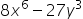.Hint:

## The correct answer is: polynomial

### Step 1 of 2:Factoring a polynomial is the process of decomposing a polynomial into a product of two or more polynomials. This can be done with the help of identities, which would speed up the process and make it simple.The given polynomial is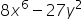. It can be written as: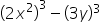here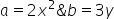.Step 2 of 2:Substitute the values ofin the identity;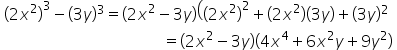Thus, the factor is: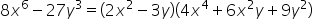We use identities to speed up the process of multiplication and simplification. There are some basic polynomial identities that you need to by heart.#### With Turito Foundation.#### Get an Expert Advice From Turito.